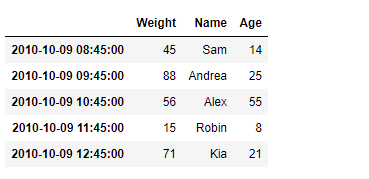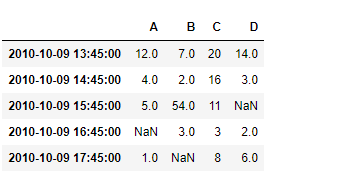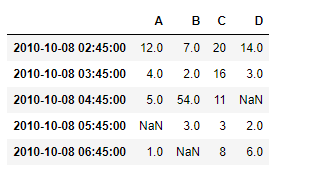Open in App
Not now

# Python | Pandas DataFrame.tshift

• Last Updated : 21 Feb, 2019

Pandas DataFrame is a two-dimensional size-mutable, potentially heterogeneous tabular data structure with labeled axes (rows and columns). Arithmetic operations align on both row and column labels. It can be thought of as a dict-like container for Series objects. This is the primary data structure of the Pandas.

Pandas` DataFrame.tshift()` function is used toShift the time index, using the index’s frequency if available in the given dataframe.

Syntax: DataFrame.tshift(periods=1, freq=None, axis=0)

Parameter :
periods : Number of periods to move, can be positive or negative
freq : Increment to use from the tseries module or time rule (e.g. ‘EOM’)
axis : Corresponds to the axis that contains the Index

Returns : shifted : NDFrame

Example #1: Use `DataFrame.tshift()` function to shift the Datetime based Index of the given dataframe by 5 hours.

 `# importing pandas as pd ` `import` `pandas as pd ` ` `  `# Creating the DataFrame ` `df ``=` `pd.DataFrame({``'Weight'``:[``45``, ``88``, ``56``, ``15``, ``71``], ` `                   ``'Name'``:[``'Sam'``, ``'Andrea'``, ``'Alex'``, ``'Robin'``, ``'Kia'``], ` `                   ``'Age'``:[``14``, ``25``, ``55``, ``8``, ``21``]}) ` ` `  `# Create the index ` `index_ ``=` `pd.date_range(``'2010-10-09 08:45'``, periods ``=` `5``, freq ``=``'H'``) ` ` `  `# Set the index ` `df.index ``=` `index_ ` ` `  `# Print the DataFrame ` `print``(df) `

Output :Now we will use `DataFrame.tshift()` function to shift the Datetime based Index of the given dataframe by 5 hours. We will pass ‘5H’ as the freq value to the function.

 `# Shift by 5 hours ` `result ``=` `df.tshift(freq ``=` `'5H'``) ` ` `  `# Print the result ` `print``(result) `

Output :As we can see in the output, the `DataFrame.tshift()` function has successfully shifted the Datetime based index of the given dataframe by the specified frequency.

Example #2 : Use `DataFrame.tshift()` function to shift the Datetime based Index of the given dataframe by -30 periods.

 `# importing pandas as pd ` `import` `pandas as pd ` ` `  `# Creating the DataFrame ` `df ``=` `pd.DataFrame({``'Weight'``:[``45``, ``88``, ``56``, ``15``, ``71``], ` `                   ``'Name'``:[``'Sam'``, ``'Andrea'``, ``'Alex'``, ``'Robin'``, ``'Kia'``], ` `                   ``'Age'``:[``14``, ``25``, ``55``, ``8``, ``21``]}) ` ` `  `# Create the index ` `index_ ``=` `pd.date_range(``'2010-10-09 08:45'``, periods ``=` `5``, freq ``=``'H'``) ` ` `  `# Set the index ` `df.index ``=` `index_ ` ` `  `# Print the DataFrame ` `print``(df) `

Output :Now we will use `DataFrame.tshift()` function to shift the Datetime based Index of the given dataframe by -30 periods. This will shift the index on the same frequency by 30 periods in the past.

 `# Shift by -30 periods ` `result ``=` `df.tshift(periods ``=` `-``30``) ` ` `  `# Print the result ` `print``(result) `

Output :As we can see in the output, the `DataFrame.tshift()` function has successfully shifted the Datetime based index of the given dataframe by the specified periods.

My Personal Notes arrow_drop_up
Related Articles